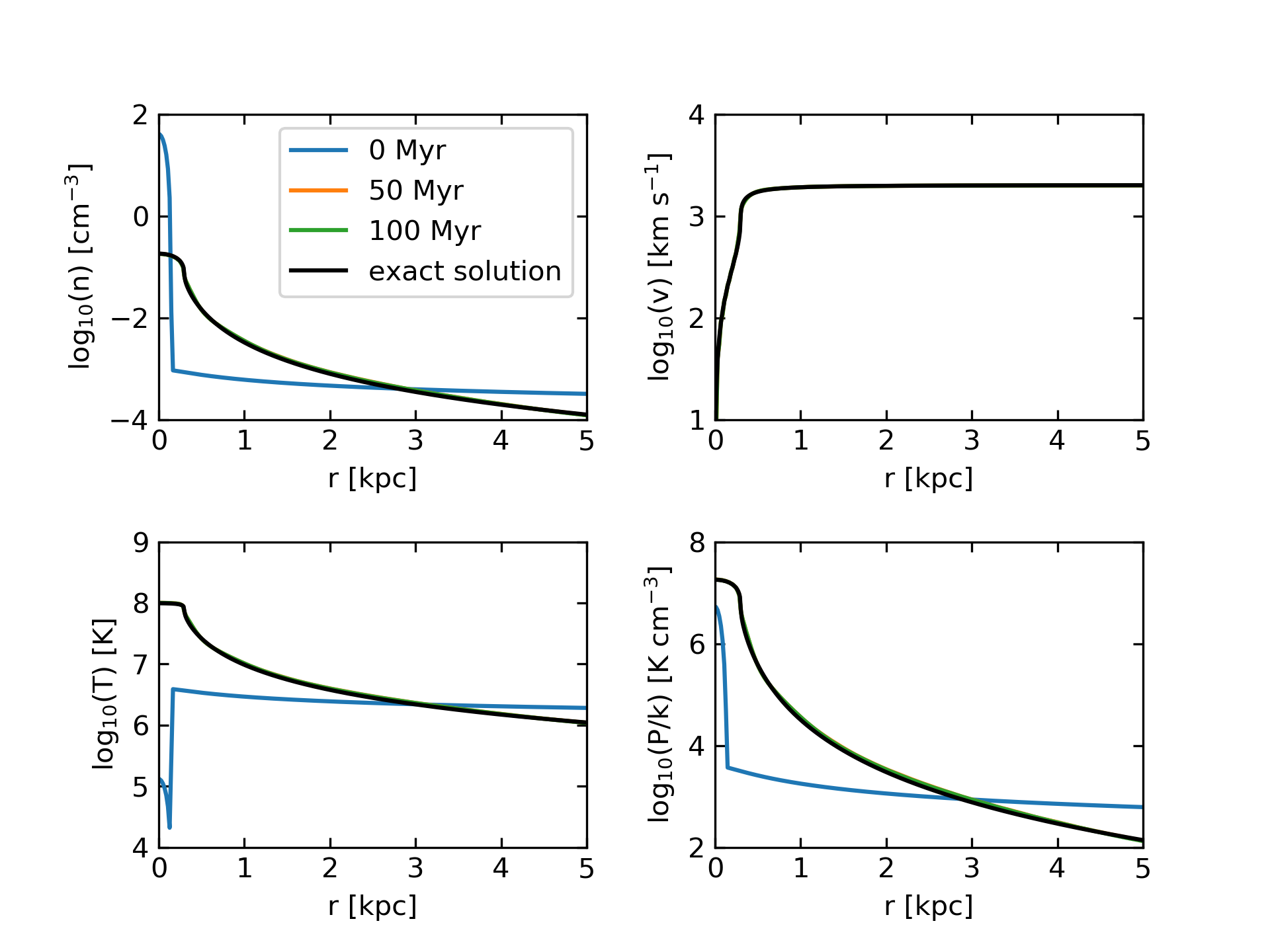# CC85 outflow (for real)

## June 19, 2017

A lot has happened in the last 4 days. To quickly summarize:

• Once I started injecting the correct amount of mass and energy, the code started breaking.
• I implemented a first-order flux correction to deal with the negative pressures.
• Combined with the dual-energy scheme, the first order flux correction works!
• I’ve successfully run several models of M82 with a Chevalier & Clegg style outflow.
• The simulations are awesome.

I’m dedicating a quick separate post to the first-order flux correction, so I’ll skip those bullet points for now. The important part is that the fix seems pretty robust, and so far, has been able to completely eliminate negative temperatures and densities in the simulation. I haven’t yet tested it with cooling…

The Simulation

Using the same M82 setup that I’ve described previously, I’ve now successfully run three outflow simulations, with the resolution going from $128^3$ (78 pc) to $512^3$ (19.5 pc). I’m currently running a $1024^3$ simulation, but having weird I/O issues on Titan that are holding things up. Movies showing the projected density, projected temperature, and temperature slices of the $512^3$ run are below. For the feedback, I used parameters near the middle of the range of acceptable fits in Strickland & Heckman 2009. They are $R_{*} = 300$pc, $\dot{M}_{\odot} = 2 M_{\odot}$/yr, and $\dot{E} = 2.6\times10^{42}$erg/s. This corresponds to $\beta = 1.4$, $\alpha = 0.9$, and uses their assumed star formation rate of 4.7 $M_\odot$/yr.

$512^3$ density projection:

$$512^3$$ temperature projection:
$$512^3$$ temperature slices:

I haven’t had time to do a whole lot of analysis, but I did check how well the outflow matches the analytic model along the z-axis, where it looks relatively unperturbed. The result is: strikingly well. Here’s a plot showing various physical parameters with both the simulation data and the analytic solution:Once the outflow is established, the simulation data is very stable along the z-axis (which is why you can’t even see the 50 Myr line), and the match with the CC85 analytic solution is spot on.